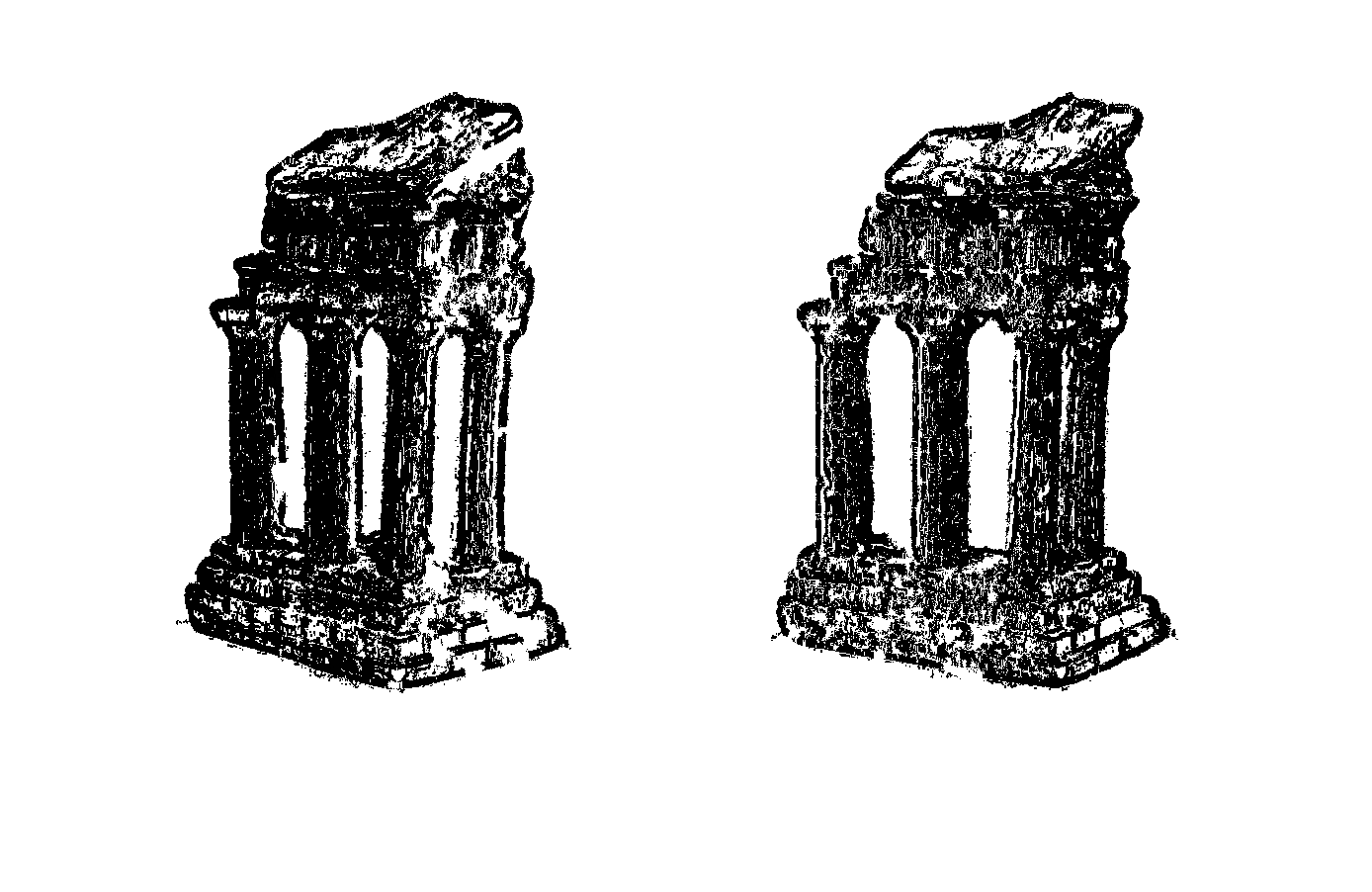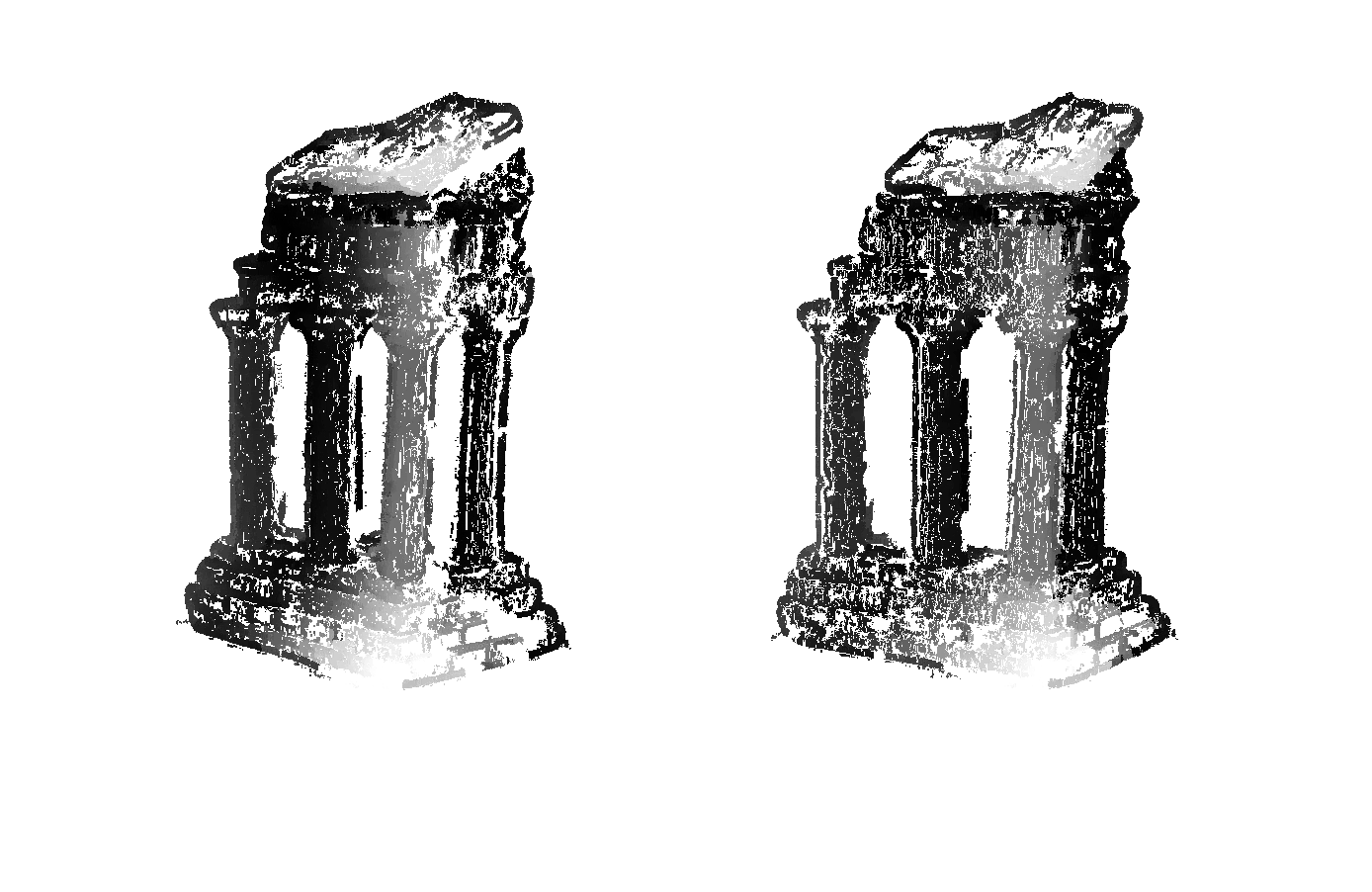# 3d Reconstruction (from 2d Images)

Does the power of reconstructing 3d scenarios from 2d images fascinate you? Back in around 2009, several people from Google, Microsoft Research and University of Washington did a research project together called Building Rome in a Day. That fascinated me inside out.

It just looked like magic and soon I got addicted to Computer Vision. Soon I noticed that 3d reconstruction is a classic CV problem and I should be able to do similar things. During the 2nd hard of the semester, I did a small experiment of 3d reconstruction (from 2d images) as the final project for my elective Computer Vision at Carnegie Mellon University, using MATLAB, c++ and openGL.

The stl model above is the reconstruction of the following images.

Here is the high-level abstract approach I achieved it.

1. detect interesting points in all images by descriptor Binary Robust Independent Elementary Features (BRIEF)
2. find the correspondence between these interesting points by computing similarity
3. propagate corresponding points base on these interesting points
4. calculate 3d position of all the points
5. generate a mesh for the point cloud we have

What does a point look like for a computer? Or how to represent a point?

We represent a point by a descriptor containing information about itself and its neighbors. We can use the descriptor to compare this point to other points to find the correspondence, the similarity score between 2 points is positively correlated to the possibility of these 2 points are the same point.

A simple descriptor example could be the following

On the left we have a image marked by the yellow region, and on the right we have a matrix representation of the image.
The red matrix is a simple descriptor of the blue point.

What defines the interestingness of a point/feature of a image?

1. it has rich image content, i.e. color variations, gradient variations and etc
2. it has well defined representation thus we can easily compare it with other points to find the correspondence
3. its position is well defined in the image
4. it is (relatively) invariable to rotation, scaling and lighting condition changes

Hmmmm. Okay, it looks somethings like this:

Generally, interesting points are the “corner”s in a image.

Some of you may realize that the original images have color but the images I used to compute interesting points are gray scale images. It simply because of the efficiency, a rgb representation of a 800*600 image is a 800*600*3 matrix while a gray scale image is just a 800*600 matrix and we lost almost nothing if we only need to compute the correspondence by a descriptor based on gradient.

When we find the correspondence as the 1st step, we only keep those pair of points that we are highly confident in. To make it precise, we need the similarity of these correspondences very high, like 95%.

But there are only 181 points in the upper image, how could we make a reasonable mesh out of it? No way we can do it if we don’t propagate the correspondence.

Why we didn’t find enough correspondence in the first place? Because the rotation and transition of the camera, the object looks slightly different in different perspective. The number of correspondence can be improved by using different descriptor but there is no big difference.

I made a simple assumption for propagating the correspondence, if 2 points are the same point then there are more correspondences among their neighbors.

That said, if point A in image1 is corresponding to point B in image2, then point A’s neighbor point C’s correspondence should be located around point B.

I put all initial matches into a priority queue with their normalized similarities as the key. While the queue is not empty, pop the best match with highest score and add new matches in their spatial neighborhood into queue. And we have a more flexible threshold at here to determine correspondence, if we still use 95% we shouldn’t find any new matches.

To make up the accuracy lost by lowering the threshold, I added another constraint called Epipolar Constraint. Find more on wikipedia.

After the propagation, we got something like thiscorrespondence after propagation, from 181 points to 74492 points

Then we can start compute the 3d position of these points (note if the cameras are in parallel, changes need to be made)

X is a point on the object and leftview/righrview are 2 images from different angle.
XL/R is the projection of X on camera OL/R
OL, OR and X are co-planar on a plane called Epipolar plane.
The corresponding point of XL must be on the red line.

And we know that the Scalar Triple Product of these 3 vectors is 0.

There is another matrix calculation involved in here but it remains the same for the rest of the calculation and it only needs simple L2 minimum optimization.

So far, we already got the point cloud need to generate the mesh, the depth graph looks something like the following:depth graph (darker <=> closer to the screen)

The last step is make a mesh representation of the point cloud. I chose a technique called α-shape, you can find more on wikipedia and here.

I found a very intuitive explanation online about how it works.

Imagine a huge mass of ice-cream making up the space Rd and containing the points S as “hard” chocolate pieces. Using one of these sphere-formed ice-cream spoons with radius α we carve out all parts of the ice-cream block we can reach without bumping into chocolate pieces, thereby even carving out holes in the inside (eg. parts not reachable by simply moving the spoon from the outside). We will eventually end up with a (not necessarily convex) object bounded by caps, arcs and points. If we now straighten all “round” faces to triangles and line segments, we have an intuitive description of what is called the α-shape of S.

The size of α represent the complexity of the shape, if α is zero, then we get the point cloud, if α is infinity then we get a convex hull.

Here is a 2d example of alpha shape:

The 2nd picture above is a convex hull. And how to find the best α was a little bit tricky for me to solve at that point so I simply use my eyes to evaluate the α.

The mini steps for the final step
1. Delaunay triangulation
2. remove tiny volume tetrahedral and check whether we can fit in a sphere with radius alpha
3. if we can, delete this tetrahedral
4. fix the holes caused by the deletion in step3

And that is all.

At this point, I didn’t know how to map the texture to the mesh (and the object itself don’t have complex texture).

After several semesters, I made a textured 3d reconstruction from multiple images (much greater than 2) and I cleaned it up in Autodesk MAYA.

Here is what is looks like

Thanks for your attention.